### Support Vector Machine Tutorial

• date 15th February, 2020 |
• by Prwatech |

# Support Vector Machine Tutorial for Beginners

Support Vector Machine Tutorial for Beginners, Are you the one who is looking forward to knowing about What is Support Vector Machine?? Or the one who is looking forward to knowing How does SVM work? and implementing svm in python or Are you dreaming to become to certified Pro Machine Learning Engineer or Data Scientist, then stop just dreaming, get your Data Science certification course with Machine Learning from India’s Leading Data Science training institute.

Support Vector Machine is another simple algorithm that every machine learning expert uses. It is highly preferred by many experts because it provides accurate results with less computation power and is used for both classification and regression problems. In this blog, we will learn How does SVM work in Machine Learning and implementing svm in python. Do you want to know What is Support Vector Machine, So follow the below mentioned support vector machine tutorial for beginners from Prwatech and take advanced Data Science training with Machine Learning like a pro from today itself under 10+ Years of hands-on experienced Professionals.

## Introduction to Support Vector Machine

Support Vector Machine (SVM) is a supervised machine learning algorithm which is like a discriminative classifier defined by a separating hyperplane. Means, for labeled training data the algorithm designs the best hyperplane which categorizes new inputs. The hyperplane in two-dimension is a line dividing space into two parts.  Support Vectors are the co-ordinates of distinct observations.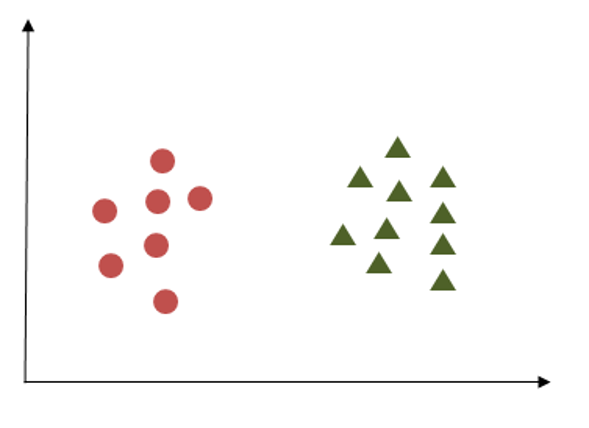## How does the Support Vector Machine work?

There is a number of possible hyperplanes that could be chosen to separate the two classes of data points. The hyperplane having a maximum margin must be chosen. Here maximum margin means the maximum distance between closest data points of both classes. To identify the accurate hyperplane there are some criteria.

### Criterion 1

The following image shows three hyperplanes trying to separate out two classes.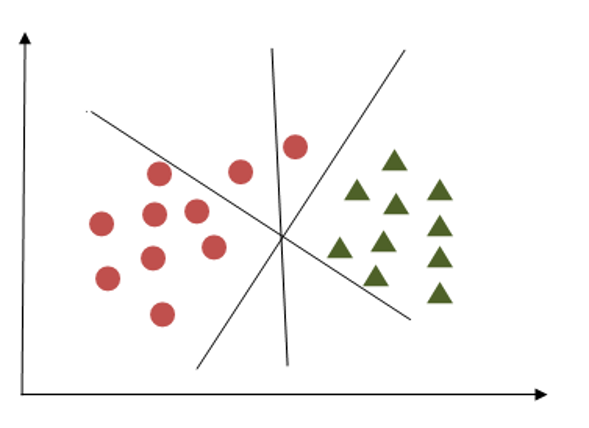Here we have to choose that hyperplane which segregates two classes. We can see hyperplane X fulfills this criterion.

### Criterion 2

Here all hyperplanes are separating two classes, now the question is how to identify the correct one?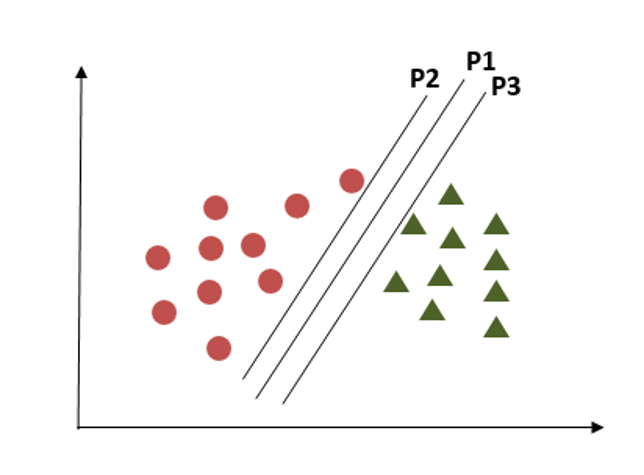Here we have to consider the maximum distance between the nearest data points in both classes and the hyperplane. This distance is called ‘Margin’. In the above diagram plane, P1 is having maximum distance from the nearest points in both classes.

### Criterion 3In this criterion, if we choose hyperplane P2 according to a higher margin than P1, it misclassified the data points. So, hyperplane P2 has classification errors but hyperplane P1 can classify correctly.

### Criterion 4: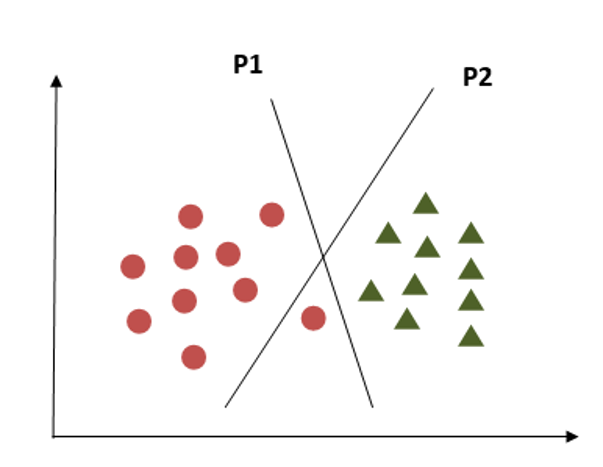What if the classes are distributed as shown in the above diagram? SVM has a property to ignore the outliers. It is a robust algorithm in case of outliers.

### Criterion 5: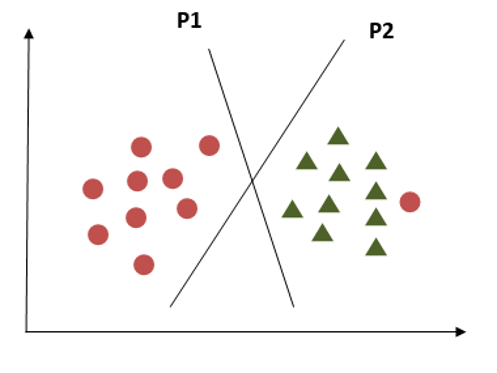Now how to handle this criterion, this is a challenge in using a single line as a hyperplane. SVM handles this problem by using additional features. It can use third plane Z, besides X and Y planes having equation like

z = x^2+y^2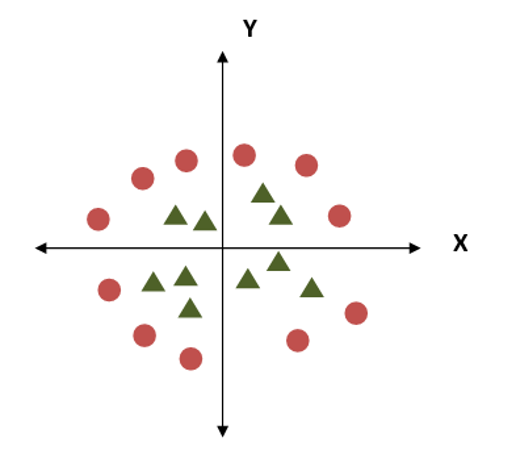AS we plot the data points across X-Z planes we get the above diagram which clearly shows the segregation of two classes. SVM can handle the separation of different types of data points with appropriate hyperplanes. In the SVM model some parameters are required defined to be tuned for efficient working of that model.

### Tuning parameters

#### Kernel:

It is important to factor that decides the nature of hyperplane. Different types of kernels present in model design. In Linear Kernel, the equation for prediction of a new data points is done by the dot product between the input (x). In this each support vector (Xi) is calculated as follows:

f(x) = B(0) + sum(ai * (x,Xi))

The equation calculates the inner products of a new input vector x with all support vectors in training data. B0 and ai coefficients for, each input, must be assessed from the training data by learning algorithm.  The polynomial kernel can be written as

K(x,xi) = 1 + sum(x * Xi)^d

And exponential can be written as

K(x,xi) = exp(-gamma * sum((x — xi²))

#### Regularization

The Regularization parameter is also called parameter ‘C’ in sklearn library. It is helpful in SVM optimization to decide to what extent the misclassification can be avoided.

For greater values of C, a smaller-margin hyperplane is selected by the optimizer, if that hyperplane performs better in classifying all the training points correctly. Whereas, for a smaller value of C the optimizer will select a larger-margin separating hyperplane, even if that hyperplane misclassified more points.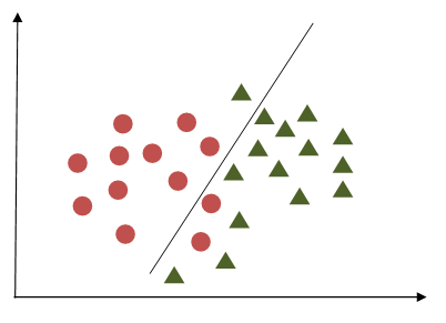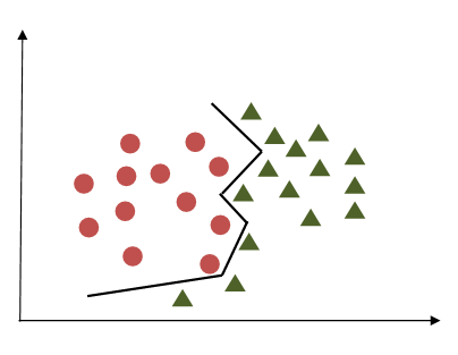The first image shows the case of lower regularization where chances of misclassifications are more. In second image high regularization will help to classify data points correctly compared to the first case.

#### Gamma

The gamma parameter defines how far the impact of a single training point reaches, with low values (meaning ‘far’) and high values (meaning ‘close’). In other words, with low gamma, points far away from reasonable separation line are taken in the calculation for the separation line. And in case of higher gamma value, the points close to the plausible line are taken in the calculation.

#### Margin

A margin is a separation of the line to the nearest points of classes. For a good Margin, the separation is larger for both classes. A good margin permits the points to be in their individual classes without crossing other classes. Let’s see an example of it.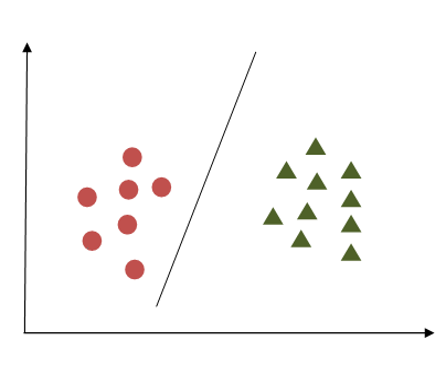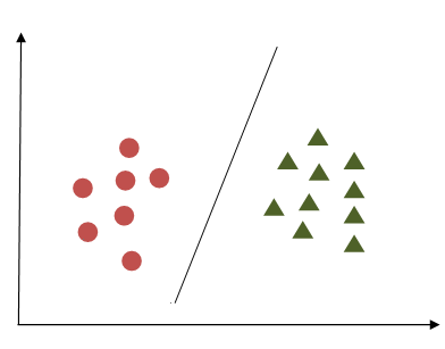In the first example, we can see the margin is not proper as it is very close to first-class having circles. But in the second example, the margin is good as it maintains a larger distance from the nearest points in both classes.

### Support Vector Machine Example

In the first example, we can see the margin is not proper as it is very close to first-class having circles. But in the second example, the margin is good as it maintains a larger distance from the nearest points in both classes.

Let’s see how we can implement this in python and what are the effects of different parameters on the result: For this, we will take data set from scikit library.

### Initializing and importing required libraries

import NumPy as np

import matplotlib.pyplot as plt

from sklearn import svm, datasets

### Let’s take the iris data set and load it. We will check features in iris and we will select two features from them to avoid complex visualization.

print(iris.feature_names)

Xin = iris.data[:, :2] # we will take ‘sepal width’ and ‘sepal length’.

yin = iris.target

We will try to plot support vectors using linear kernel, polynomial kernel, and sigmoid kernel. For this we will keep C and gamma values constant.

### Let’s apply the linear kernel first:

C = 1.0 # Support Vector Machine regularization parameter

svc = svm.SVC(kernel=’linear, C=1,gamma=’auto’).fit(X, y)

x_min, x_max = Xin[:, 0].min() – 1, Xin[:, 0].max() + 1

y_min, y_max = Xin[:, 1].min() – 1, Xin[:, 1].max() + 1

h = (x_max / x_min)/100

x1, y1 = np.meshgrid(np.arange(x_min, x_max, h),np.arange(y_min, y_max, h))

plt.subplot(1, 1, 1)

Z = svc.predict(np.c_[x1.ravel(), y1.ravel()])

Z = Z.reshape(x1.shape)

plt.contourf(x1, y1, Z, cmap=plt.cm.Paired, alpha=0.8)

plt.scatter(Xin[:, 0], Xin[:, 1], c=yin, cmap=plt.cm.Paired)

plt.xlabel(‘Sepal length’)

plt.ylabel(‘Sepal width’)

plt.xlim(x1.min(), x1.max())

plt.title(‘SVC – linear kernel’)

plt.show()

### Output: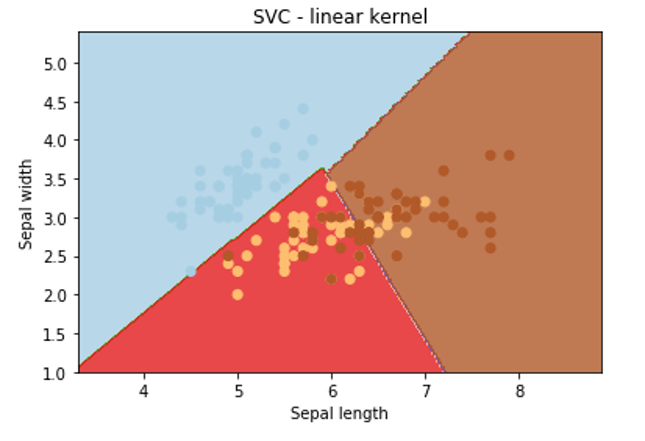Now we have to see the results of different kernels. By keeping rest code similar we have to just change the type of kernel as follows

### Let’s apply poly kernel:

svc = svm.SVC(kernel=’poly’, C=1,gamma=’auto’).fit(X, y)

### Output: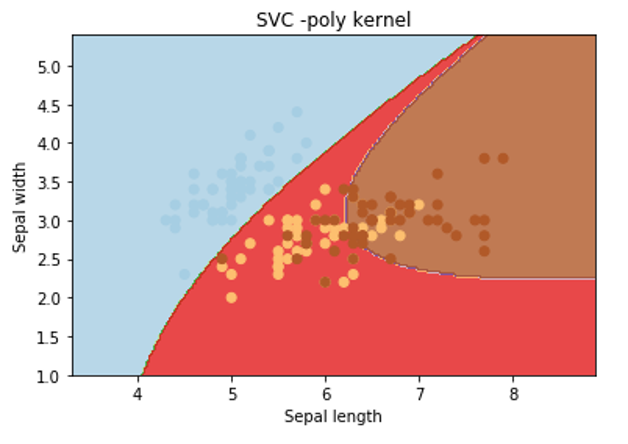### Let’s apply rbf kernel:

svc = svm.SVC(kernel=’rbf’, C=1,gamma=’auto’).fit(X, y)

### Output: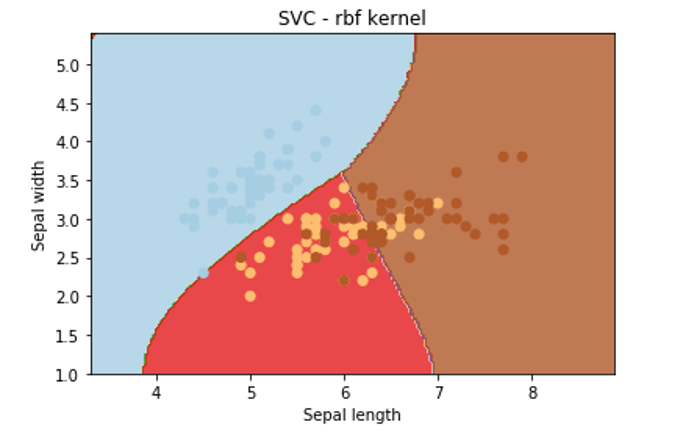From the above graphical representation, it is clearly observed that change in the kernel will change the contour in the image and try to classify data points in a different manner to approach the correct classification.

### Now Let’s observe the effect of ‘gamma’ on classification. For that, we will keep the SVM kernel and C constant.

If we change values of gamma as 1,10,200 then we get the respective graph as follows: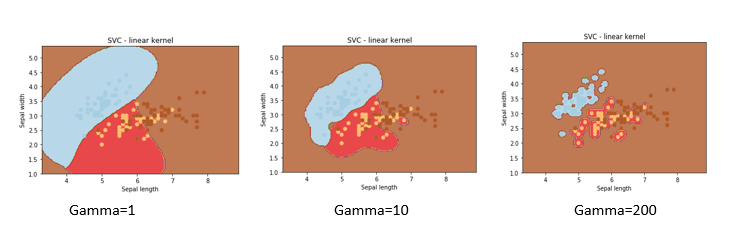### Now Let’s observe the effect of ‘C’ on classification. For that, we will keep gamma and SVM kernel constant.

If we change values of C as 5,500,5000 then we get the respective graph as follows: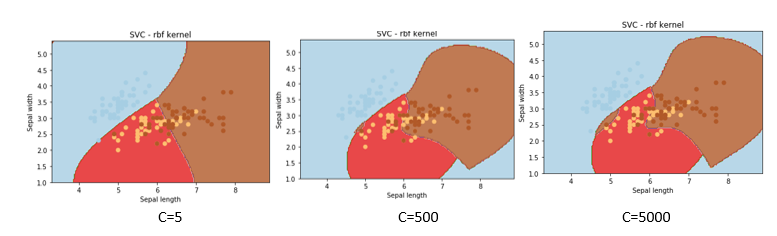We hope you understand the support vector machine tutorial for beginners. Get success in your career as a Data Scientist by being a part of the Prwatech, India’s leading Data Science training institute in Bangalore.

### Quick Support